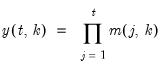Command Reference : Matrix Language Reference

 @colcumprod
Compute cumulative products for each column of a matrix.
Syntax: @colcumprod(m)
m: matrix, vector
Return: matrix, vector
Returns a matrix where each column contains the cumulative products of the values of the corresponding column of m.
For each element of the output, compute the cumulative product of the values in m from the start of the column up to the current row:Note that this function is prone to numeric overflow.
Examples
matrix m1 = @mnrnd(10, 3)
matrix cumprod = @colcumprod(m1)
computes the cumulative product for each column of matrix M1 and places the results in CUMPROD.
Cross-references